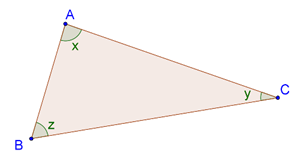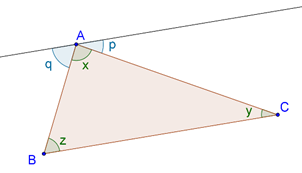# Proof of the Angle Sum Property

Proof of the Angle Sum Property

Why should the angles in any triangle sum to 1800? This question is answered by the proof of the angle sum property, which we are going to discuss now.

Consider an arbitrary triangle, $$\Delta {\rm{ABC}}$$, as shown below:We have to show that the sum of the angles x, y and z is 1800. We draw a line L through the vertex A, which is parallel to the side BC, as shown below:Two additional angles are formed, which we have marked p and q. Now, we proceed to the proof.

Proof: Since AB is a transversal for the parallels L and BC, we have

p = y (alternate interior angles)

Similarly,  q = z. Now, p, x and q must sum to 1800 (why):

p + x + q = 1800

è y + x + z = 1800

Thus, the sum of the three angles x, y and z is 1800. And it should be obvious that this will hold true for any triangle, since the same proof is valid for any arbitrary triangle.

Geometry
grade 9 | Questions Set 1
Geometry
Geometry
grade 9 | Questions Set 2
Geometry
More Important Topics
Numbers
Algebra
Geometry
Measurement
Money
Data
Trigonometry
Calculus
More Important Topics
Numbers
Algebra
Geometry
Measurement
Money
Data
Trigonometry
Calculus